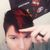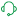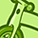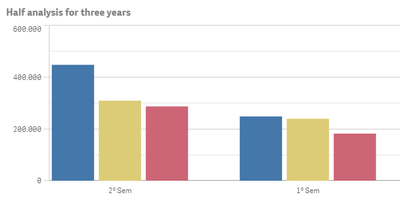# Knowledge Base

Search or browse our knowledge base to find answers to your questions ranging from account questions to troubleshooting error messages. The content is curated and updated by our global Support team

Announcements
Live chat with experts, bring your API Integration questions. June 15th, 10 AM ET. REGISTER TODAY

# How to create on your own an Autocalendar (Derived fields)Digital Support

## How to create on your own an Autocalendar (Derived fields)

The information and script in this article are provided as is. For further customization and assistance, engage with our active QlikView or Qlik Sense app-building boards.

If you have a group of fields that are related, or if fields carry information that can be broken up into smaller parts that are relevant when creating dimensions or measures, you can create field definitions that can be used to generate derived fields. One example is a date field, from which you can derive several attributes, such as year, month, week number, or day name. All these attributes can be calculated in a dimension expression using Qlik Sense date functions, but an alternative is to create a calendar definition that is common for all fields of date type. Field definitions are stored in the data load script.

Note in case that you need to change the format / to customize the date you need to do it from the script, and most probably drill down to all levels, depending of course the object and what you want to accomplish.

Here is such an example of an autocalendar that can be used in any case:

``````[autoCalendar]:
DECLARE FIELD DEFINITION Tagged ('\$date')
FIELDS
Dual(Year(\$1), YearStart(\$1)) AS [Year] Tagged ('\$axis', '\$year'),
Dual('Q'&Num(Ceil(Num(Month(\$1))/3)),Num(Ceil(NUM(Month(\$1))/3),00)) AS [Quarter] Tagged ('\$quarter', '\$cyclic'),
Dual(Year(\$1)&'-Q'&Num(Ceil(Num(Month(\$1))/3)),QuarterStart(\$1)) AS [YearQuarter] Tagged ('\$yearquarter', '\$qualified'),
Dual('Q'&Num(Ceil(Num(Month(\$1))/3)),QuarterStart(\$1)) AS [_YearQuarter] Tagged ('\$yearquarter', '\$hidden', '\$simplified'),
Month(\$1) AS [Month] Tagged ('\$month', '\$cyclic'),
Dual(Year(\$1)&'-'&Month(\$1), monthstart(\$1)) AS [YearMonth] Tagged ('\$axis', '\$yearmonth', '\$qualified'),
Dual(Month(\$1), monthstart(\$1)) AS [_YearMonth] Tagged ('\$axis', '\$yearmonth', '\$simplified', '\$hidden'),
Dual('W'&Num(Week(\$1),00), Num(Week(\$1),00)) AS [Week] Tagged ('\$weeknumber', '\$cyclic'),
Date(Floor(\$1)) AS [Date] Tagged ('\$axis', '\$date', '\$qualified'),
Date(Floor(\$1), 'D') AS [_Date] Tagged ('\$axis', '\$date', '\$hidden', '\$simplified'),
If (DayNumberOfYear(\$1) <= DayNumberOfYear(Today()), 1, 0) AS [InYTD] ,
Year(Today())-Year(\$1) AS [YearsAgo] ,
If (DayNumberOfQuarter(\$1) <= DayNumberOfQuarter(Today()),1,0) AS [InQTD] ,
4*Year(Today())+Ceil(Month(Today())/3)-4*Year(\$1)-Ceil(Month(\$1)/3) AS [QuartersAgo] ,
Ceil(Month(Today())/3)-Ceil(Month(\$1)/3) AS [QuarterRelNo] ,
If(Day(\$1)<=Day(Today()),1,0) AS [InMTD] ,
12*Year(Today())+Month(Today())-12*Year(\$1)-Month(\$1) AS [MonthsAgo] ,
Month(Today())-Month(\$1) AS [MonthRelNo] ,
If(WeekDay(\$1)<=WeekDay(Today()),1,0) AS [InWTD] ,
(WeekStart(Today())-WeekStart(\$1))/7 AS [WeeksAgo] ,
Week(Today())-Week(\$1) AS [WeekRelNo] ;

DERIVE FIELDS FROM FIELDS [Date] USING [autoCalendar] ;``````

Labels (1)
• ### App Development

CommentsPartner

Hello , how I can make half year derived in autocalendar ?

I make this:

Dual('H'&Num(Ceil(Num(Month(\$1))/6)),Num(Ceil(NUM(Month(\$1))/6),00)) AS [Half] Tagged ('\$half', '\$cyclic'),

or

'HY' & ceil(month(date#((\$1),'MMM YYYY'))/6) as HalfYear,

The derivation was created, but in the graph it does not look like the year next to it is only half.

My Script:

[Calendar]:
DECLARE FIELD DEFINITION Tagged ('\$date')
FIELDS
Dual(Year(\$1), YearStart(\$1)) AS [Ano] Tagged ('\$axis', '\$year'),
//Dual('H'&Num(Ceil(Num(Month(\$1))/6)),Num(Ceil(NUM(Month(\$1))/6),00)) AS [Half] Tagged ('\$half', '\$cyclic'),
//'HY' & ceil(month(date#((\$1),'MMM YYYY'))/6) as HalfYear,
ceil(Month(\$1)/6)&'º Sem' as [Semestre],
Dual('Q'&Num(Ceil(Num(Month(\$1))/3)),Num(Ceil(NUM(Month(\$1))/3),00)) AS [Trimestre] Tagged ('\$quarter', '\$cyclic'),
Dual(Year(\$1)&'-Q'&Num(Ceil(Num(Month(\$1))/3)),QuarterStart(\$1)) AS [Ano Trimestre] Tagged ('\$yearquarter', '\$qualified'),
Dual('Q'&Num(Ceil(Num(Month(\$1))/3)),QuarterStart(\$1)) AS [_YearQuarter] Tagged ('\$yearquarter', '\$hidden', '\$simplified'),
//Dual('H'&Num(Ceil(Num(Month(\$1))/6)),QuarterStart(\$1)) AS [_YearQuarter] Tagged ('\$yearquarter', '\$hidden', '\$simplified'),
Month(\$1) AS [Mês] Tagged ('\$month', '\$cyclic'),
Dual(Year(\$1)&'-'&Month(\$1), monthstart(\$1)) AS [Ano/Mês] Tagged ('\$axis', '\$yearmonth', '\$qualified'),
Dual(Month(\$1), monthstart(\$1)) AS [_YearMonth] Tagged ('\$axis', '\$yearmonth', '\$simplified', '\$hidden'),
Dual('W'&Num(Week(\$1),00), Num(Week(\$1),00)) AS [Semana] Tagged ('\$weeknumber', '\$cyclic'),
Date(Floor(\$1)) AS [DATA] Tagged ('\$axis', '\$date', '\$qualified'),
Date(Floor(\$1), 'D') AS [_Date] Tagged ('\$axis', '\$date', '\$hidden', '\$simplified'),
weekday(\$1) as [Dia da Semana],
Week(Today())-Week(\$1) AS [WeekRelNo] ;

DERIVE FIELDS FROM FIELDS [C5_EMISSAO] USING [Calendar] ;Its ok!

In half year:it does not look like the year next to it is only half.

HelpDigital Support

Hello @RochaPedro !

I'd recommend taking this over to the respective board for App building (Qlikview or Qlik Sense, depending on what you are working with). You'll be able to get the right eyes on your query there!

I grabbed you the links to the boards:  QlikView or Qlik Sense

Version history
Revision #:
5 of 5
Last update:
‎2021-03-16 03:24 AM
Updated by:Contributors